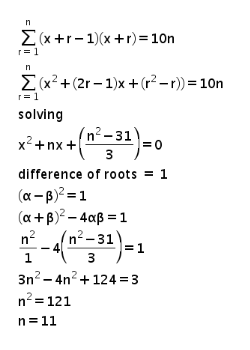# If, for a positive integer n, the quadratic equation, x(x+1)+(x+1)(x+2)+

If, for a positive integer n, the quadratic equation, x(x+1)+(x+1)(x+2)+… +( n 1) x + − (x+n)=10n has two consecutive integral solutions, then n is equal to :